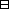Ordered Fields

## Notation

We will use the following notation. We will write

1. $a, if $a\le b$ and $a\ne b$,
2. $a\ge b$, if $a\nleqq b$ or $a=b$, and
3. $a>b$, if $a\nleqq b$,
where $a,b\in S$. Note that these definitions correspond to the usual conventions.

## Ordered fields

An ordered monoid is a commutative monoid $G$ with an ordering $\le$ such that

if $x,y,z\in G$ and $x\le y$ then $x+z\le y+z$.

An ordered group is an abelian group $G$ with an ordering $\le$ such that

if $x,y,z\in G$ and $x\le y$ then $x+z\le y+z$.

An ordered ring is a commutative ring $A$ with an ordering $\le$ such that

1. $A$ is an ordered group under $+$, and
2. if $x,y\in A$ and $x\ge 0$ and $y\ge 0$ then $xy\ge 0$.

An ordered field is a field $𝔽$ with a total ordering $\le$ such that $𝔽$ is an ordered ring.

Let $G$ be an ordered group and let $x\in G$. The element $x$ is positive if $x\ge 0$. The element $x$ is negative if $x\le 0$. The element $x$ is strictly positive if $x>0$. The element $x$ is strictly negative if $x<0$.

Let $G$ be a lattice ordered group. If $x\in G$ define

${x}^{+}=\mathrm{sup}\left\{x,0\right\}\text{,}\phantom{\rule{2em}{0ex}}$ and $\phantom{\rule{2em}{0ex}}{x}^{-}=\mathrm{sup}\left\{-x,0\right\}$

Let $G$ be a lattice ordered group and let $x\in G$. the absolute value of $x$ is

$\left|x\right|=\mathrm{sup}\left\{x,-x\right\}$.

Let $G$ be an ordered group. Let $x,y\in G$. The elements $x$ and $y$ are coprime if $\mathrm{inf}\left(x,y\right)=0$.

Let $G$ be an ordered group. Let $x\in G$. The element is irreducible if it is a minimal element of a set of strictly positive elements of $G$.

Let $𝔽$ be an ordered field. If $x\in 𝔽$ define

The nonnegative integers ${ℤ}_{\ge 0}$ with the ordering defined by

$x\le y\phantom{\rule{2em}{0ex}}$ if there is an $\phantom{\rule{2em}{0ex}}n\in {ℤ}_{\ge 0}\phantom{\rule{2em}{0ex}}$ with $\phantom{\rule{2em}{0ex}}y=x+n$,

is an ordered momoid. There is a unique extension of this ordering to $ℤ$ so that $ℤ$ is an ordered group. There is a unique extension of this ordering to $ℚ$ so that $ℚ$ is an ordered field.

We still need the proper characterisation of $ℝ$ as an ordered field that contains $ℚ$ and satisfies the least upper bound property. What is the proper uniqueness statement? Should we put Dedikind cuts here?

Let $S$ be an ordered fields and $x,y\in S$ with $x\ge 0$ and $y\ge 0$, then $x ≤ y if and only if x 2 ≤ y 2 .$Proof. Assume $x,y\in S$ and $x\ge 0$ and $y\ge 0$. To show: If $x\le y$ then ${x}^{2}\le {y}^{2}$. If ${x}^{2}\le {y}^{2}$ then $x\le y$. We have: Assume ${x}^{2}\le {y}^{2}$. Then ${y}^{2}+\left(-{x}^{2}\right)\ge {x}^{2}+\left(-{x}^{2}\right)=0$. So ${y}^{2}-{x}^{2}\ge 0$. So $\left(y-x\right)\left(y+x\right)\ge 0$. Since $x\ge 0$ and $y\ge 0$ then $x+y\ge 0$ and ${\left(x+y\right)}^{-1}>0$ (or $x=0$ and $y=0$). So $\left(y-x\right)\left(y+x\right){\left(x+y\right)}^{-1}\ge 0$. So $y-x\ge 0$. Assume $y\ge x$. Then $y-x\ge 0$ Then $\left(y-x\right)\left(y+x\right)\ge 0\left(x+y\right)$. So ${y}^{2}-{x}^{2}\ge 0$. So ${y}^{2}\ge {x}^{2}$. Note: The proof above slips lots of steps. For example, the assertion that ${y}^{2}+\left(-{x}^{2}\right)=\left(y+\left(-x\right)\right)\left(y+x\right)$ (which relies on the claim that $\left(-x\right)y=-\left(xy\right)$) requires some justification.

## Exercises

 Show that if $x,y\in ℚ$ then $x\le y$ if and only if $y-x\ge 0$.